用粒子动画来忆起你的春节时光 | 支持表情文字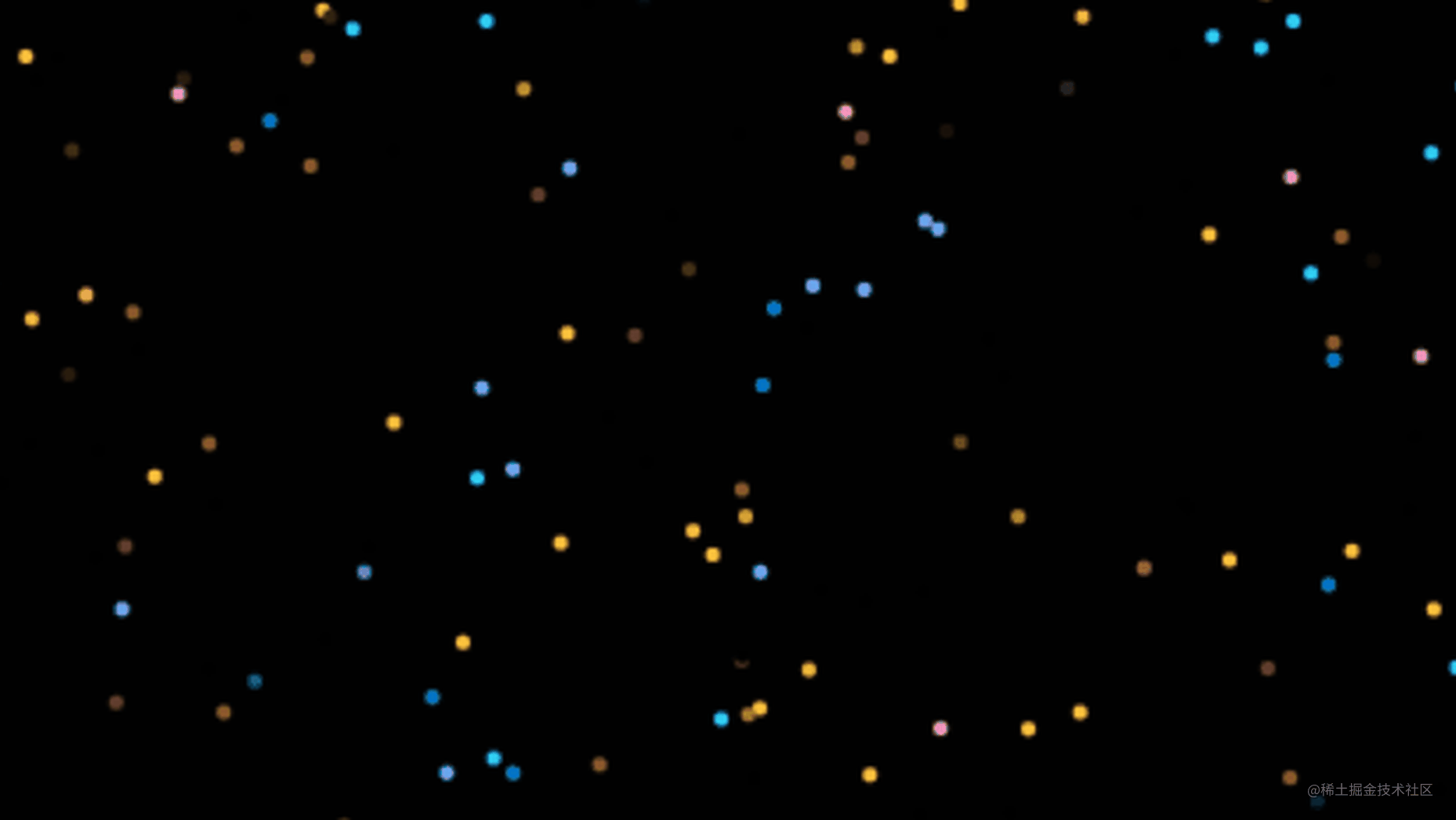PK创意闹新春，我正在参加「春节创意投稿大赛」，详情请看：春节创意投稿大赛

玩法

• 关键词输入在下划线位置
• 多个关键词可通过空格或者中英文逗号分隔
• 支持中英文、表情文字
• 文字尽量不要太长，五字以内
• 输入关键词序列后，回车键开启动画

童年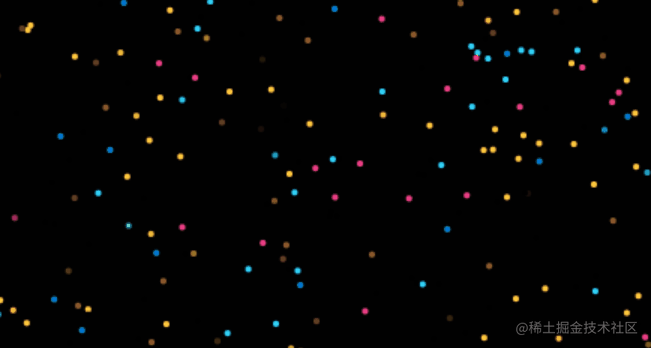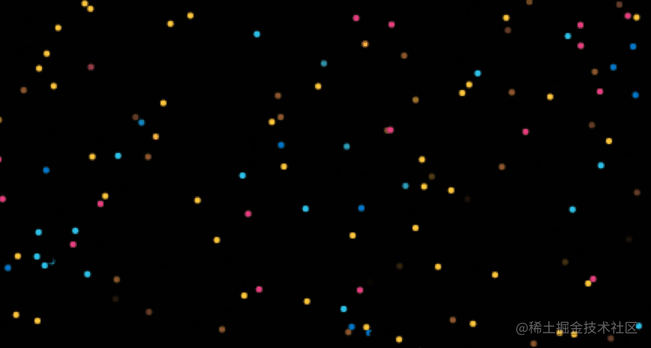青春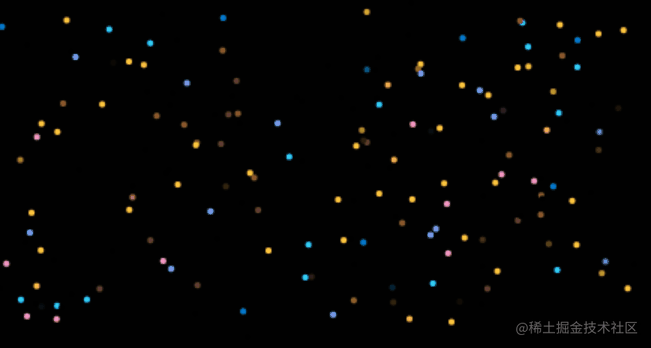疫情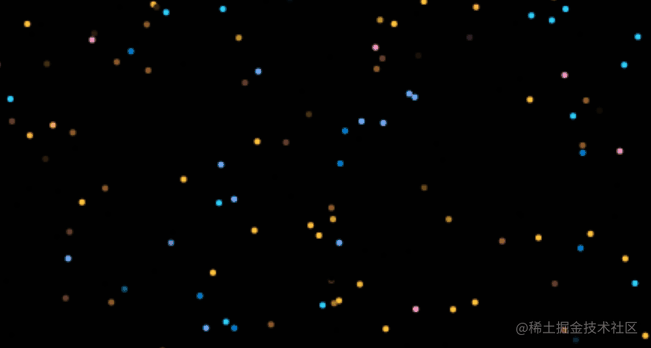代码实现

创建粒子类

class Particle {
constructor({ x = 0, y = 0, tx = 0, ty = 0, radius = 2, color = "#F00000" }) {
// 当前坐标为随机生成坐标
this.x = particle.x;
this.y = y;
// 目标点坐标(副画布原有粒子坐标)
this.tx = tx;
this.ty = ty;
this.color = color;
}
// 粒子绘制
draw(ctx) {
ctx.save();
ctx.translate(this.x, this.y);
ctx.fillStyle = this.color;
ctx.beginPath();
// 绘制圆形
ctx.arc(0, 0, this.radius, 0, Math.PI * 2, true);
ctx.closePath();
ctx.fill();
ctx.restore();
return this;
}
}

提取像素信息

创建副画布及绘制文字

const canvas = document.createElement("canvas");
const ctx = canvas.getContext("2d");
const viewWidth = window.innerWidth * 0.5;
const viewHeight = window.innerHeight * 0.5;

canvas.width = viewWidth;
canvas.height = viewHeight;
// 预留处理图片的接口
if (typeof target === "string") {
// 绘制文字
// 保证长文字的在PC端及移动端的完整展示
// 处理的有几分粗糙
ctx.font = `\${
target.length < 3 ? textWidth : (textWidth * 3) / (1 + target.length)
}px bold`;
// 设置文字的基本样式
ctx.fillStyle = colorList[rand(0, colorList.length)];
ctx.textBaseline = "middle";
ctx.textAlign = "center";
ctx.fillText(target, viewWidth / 2, viewHeight / 2);
}

获取像素点数据

• 语法 ctx.getImageData(sx, sy, sw, sh);

• 参数

• sx, sy: 要提取图像的左上角 x y 坐标
• sw, sh: 要提取图像的宽高
• 返回值

返回 ImageData 对象，该对象拷贝了指定图像区域的像素。对于图像中每个像素点，都分别存放 RGBA 四方面的信息，所有的像素数据以一维数组形式存放在 data 属性中(每个像素点由 rgba 四个值组成，因此每次需要乘以 4 才能跳到下一个像素点)

const { data, width, height } = ctx.getImageData(0, 0, viewWidth, viewHeight);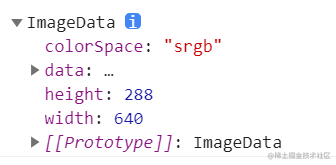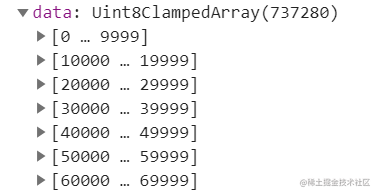提取绘制粒子的像素点

getImageData 提取画布的全部像素点，如上图 data 所示，一共提取了 737280 / 4 = 184320 个像素点，如果不经筛选全部绘制成半径为 2 的粒子，一方面粒子会发生重叠；另一方面如果生成 18万 个粒子，为了保证粒子运动的流畅性，使用 requestAnimationFrame 更新粒子位置，如此巨大数量的粒子频繁渲染对电脑的渲染性能要求极高，就比如小包的电脑，就完全无法运行，卡顿感爆棚。

// 获取目标对象的像素点，interval 控制像素点数量，值越大返回的像素点越少
const pixeles = [];
// 遍历像素数据，用interval减少取到的像素数据
for (let x = 0; x < width; x += interval) {
for (let y = 0; y < height; y += interval) {
const pos = (y * width + x) * 4;
// 只提取 rgba 中透明度大于0.5的像素，即aplha > 128
if (data[pos + 3] > 128) {
pixeles.push({
x,
y,
rgba: [data[pos], data[pos + 1], data[pos + 2], data[pos + 3]],
});
}
}
}
return pixeles;
}

绘制粒子

function createParticles({ text, radius, interval }) {
const pixeles = getWordPxInfo(text, interval);
return pixeles.map((particle) => {
return new Particle({
x: Math.random() * (50 + window.innerWidth * 0.5) - 50,
y: Math.random() * (50 + window.innerHeight * 0.5) - 50,
tx: particle.x,
ty: particle.y,
color: particle.rgba,
});
});
}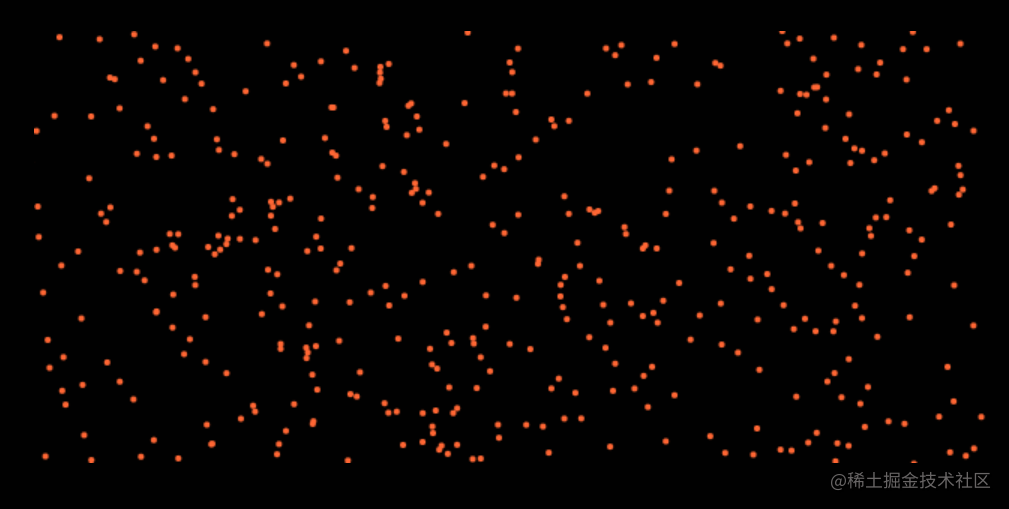文字切换

// 参数分别是粒子数组及下一个文字动画回调
function drawFrame(particles, finished) {
// 开启粒子渲染动画
const timer = window.requestAnimationFrame(() => {
drawFrame(particles, finished);
});
ctx.clearRect(0, 0, canvas.width, canvas.height);
// 缓动系数设置为0.09
const easing = 0.09;
const finishedParticles = particles.filter((particle) => {
// 当前坐标和目标点之间的距离
const dx = particle.tx - particle.x;
const dy = particle.ty - particle.y;
// 粒子移动速度
let vx = dx * easing;
let vy = dy * easing;

// 判断当前粒子是否完成动画
if (Math.abs(dx) < 0.1 && Math.abs(dy) < 0.1) {
// 完成动画位置不会在改变，并修改 finished 为 true
particle.finished = true;
particle.x = particle.tx;
particle.y = particle.ty;
} else {
// 未完成动画继续更新粒子位置
particle.x += vx;
particle.y += vy;
}
// 绘制粒子新位置
particle.draw(ctx);
// 判断返回完成运动的的粒子数
return particle.finished;
});

if (finishedParticles.length === particles.length) {
// 全部粒子运动结束，结束当前动画
window.cancelAnimationFrame(timer);
// 开启下一轮文字回调
finished && finished();
}
return particles;
}

动画切换函数 loop

loop 函数设计为了给 drawFrame 提供下一个文字的渲染。代码逻辑比较简单，不在多言。

function loop(words, i = 0) {
return drawFrame(
// 生成粒子
createParticles({ text: words[i], radius: 2, interval: 5 }),
// finish函数部分=》下一个文字
() => {
i++;
// hack一下空文字
if (i < words.length && words[i].length > 0) {
loop(words, i);
}
}
);
}

虎年送大礼

1. 小包预留俩组关键词，猜测最贴切的掘友，小包会送上掘金周边一份
2. 分享你的新春回忆(录制动图或者关键词都可)，收获到点赞和评论之后最多的掘友，小包同样也会送上掘金周边一份
3. 别的奖项暂时还没想出，后面如果想出在动态添加上